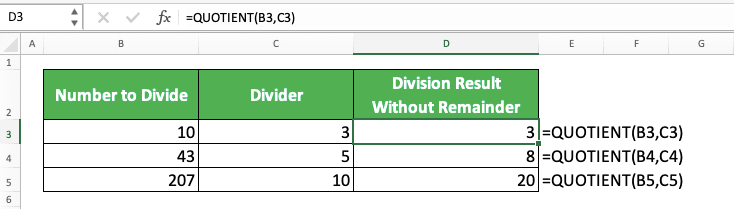How to Use the QUOTIENT Formula in Excel: Functions, Examples, and Writing Steps - Compute Expert

# How to Use the QUOTIENT Formula in Excel: Functions, Examples, and Writing Steps

Home >> Excel Tutorials from Compute Expert >> Excel Formulas List >> How to Use the QUOTIENT Formula in Excel: Functions, Examples, and Writing Steps

In this tutorial, you will learn how to use the QUOTIENT formula in excel completely.

When dividing numbers in excel, we sometimes want to have the division result without its remainder. If you use QUOTIENT, then you can get that kind of result you want easily and fast.

Disclaimer: This post may contain affiliate links from which we earn commission from qualifying purchases/actions at no additional cost for you. Learn more

## What is the QUOTIENT Formula in Excel?

QUOTIENT is a formula you can use in excel to get your number division result without remainder.

## QUOTIENT Function in Excel

You can use QUOTIENT to divide your number and get only its “clean” result without any remainder.

## QUOTIENT Result

QUOTIENT result is an integer that comes from your number division process.

## Excel Version from Which We Can Start Using QUOTIENT

We can start using QUOTIENT in excel since excel 2003.

## The Way to Write It and Its Inputs

Here is the general writing form of the QUOTIENT formula in excel.

= QUOTIENT ( number_to_divide , divider )

As you can see, the inputs you need to give to QUOTIENT while writing the formula are simple to understand. Just input the number you want to divide and the divider of that number to your QUOTIENT.

Press enter after you write it like that and you will get your number division result without a remainder!

## Example of Its Usage and Result

You can see the implementation example of QUOTIENT below.This example shows how simple it is to write QUOTIENT in excel as we previously discussed. Just input the number you want to divide and its divider to QUOTIENT to use the formula.

In the QUOTIENT results in the example, you can see that we just get our division results without their remainder. Just the results we expect to get from this formula!

## Writing Steps

Now, let’s discuss the steps to write a QUOTIENT formula in excel from the beginning until the end. These steps should be easy to follow since QUOTIENT only needs two easy-to-understand inputs.

1. Type an equal sign ( = ) in the cell where you want to put your QUOTIENT result2. Type QUOTIENT (can be with large and small letters) and an open bracket sign after =3. Input the number you want to divide. Then, type a comma sign ( , )4. Input the divider for the number you inputted previously5. Type a close bracket sign6. Press Enter
7. Done!## Why Does QUOTIENT Give Me a Wrong/Error Result? Possible Reasons & Solutions

Got a number different than what you expect from QUOTIENT or even an error? Many things can cause that to happen.

However, here we list three reasons that we think are the most possible causes and the solution for each of them. Hope they can help you to solve your QUOTIENT problem!

• Reason: You input a zero/blank cell as your number divider
Solution: Check again the divider input you give to the QUOTIENT that gives you an error result. Make sure it isn’t a zero/blank cell. Replace the divider with a number other than zero if this is indeed the reason for your QUOTIENT error

• Reason: You input data that isn’t a number into QUOTIENT
Solution: Non-number data you input into QUOTIENT will make it produces an error. If this is the case, replace your data input with a number to make your QUOTIENT function correctly

• Reason: You have a false expectation for your QUOTIENT result
Solution: Remember that QUOTIENT gives you your number division result without its remainder. If you look for something else, such as a normal division result or the division remainder, don’t use QUOTIENT

## Exercise

After you have understood how to use QUOTIENT in excel, you can practice your understanding by doing the following exercise!

### Questions

1. How many allocations of product quantities for each production machine, without decimal?
2. How many allocations of product quantities for each salesperson, without decimal?
3. How many allocations of product quantities for each distributor, without decimal?

You always get an integer when you use QUOTIENT.

Related tutorials you should learn from:

Get updated excel info from Compute Expert by registering your email. It's free!